#Function Repository Resource:

# RandomRealPoint

Randomly choose a point within a specified domain

Contributed by: Arnoud Buzing
 ResourceFunction["RandomRealPoint"][{range1,range2,…}] generates a random point in the domain defined by the ranges. ResourceFunction["RandomRealPoint"][ranges,n] generates n random points on the domain. ResourceFunction["RandomRealPoint"][ranges,{n1,n2,n3}] gives an n1× n2×… array of random points.

## Details and Options

RandomRealPoint uses RandomReal to generate points with a uniform distribution.
Possible specifications of rangei are:
 xmax between zero and xmax {xmin,xmax} in range xmin to xmax

## Examples

### Basic Examples

Find a point within a domain:

 In:=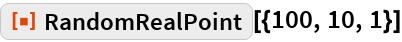Out=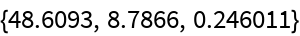Find many within a four-dimensional domain:

 In:=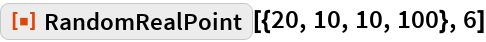Out=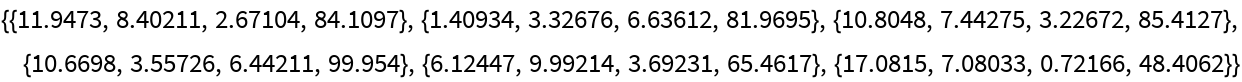Create an array of random two-dimensional points in the specified domain:

 In:=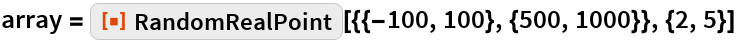Out=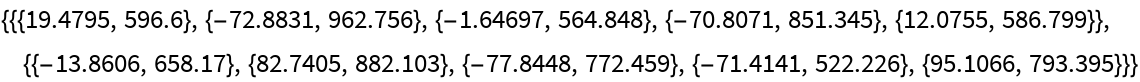Plot the points on a plane:

 In:=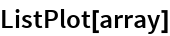Out=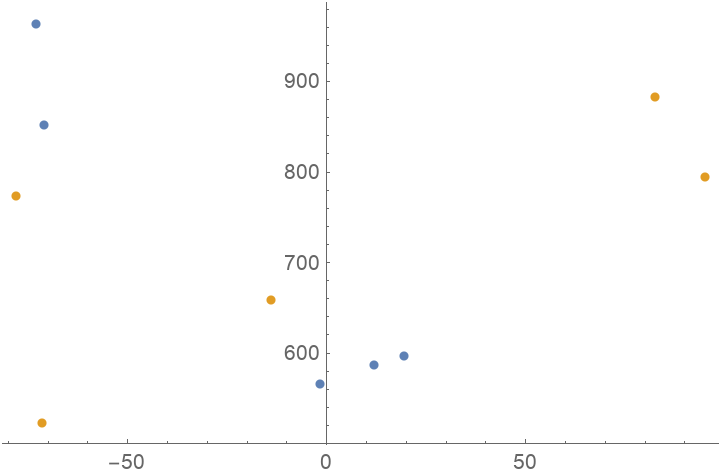### Scope

Create an array of random three-dimensional points:

 In:=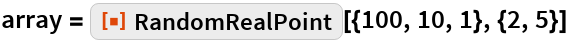Out=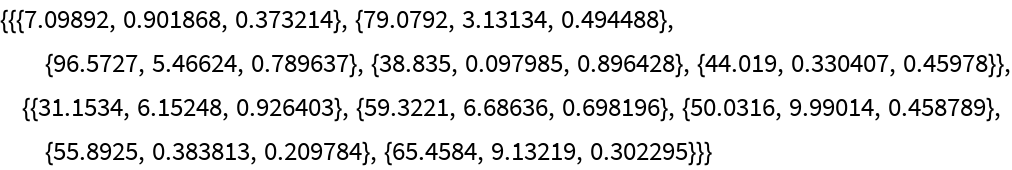Plot the points as surfaces:

 In:=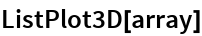Out=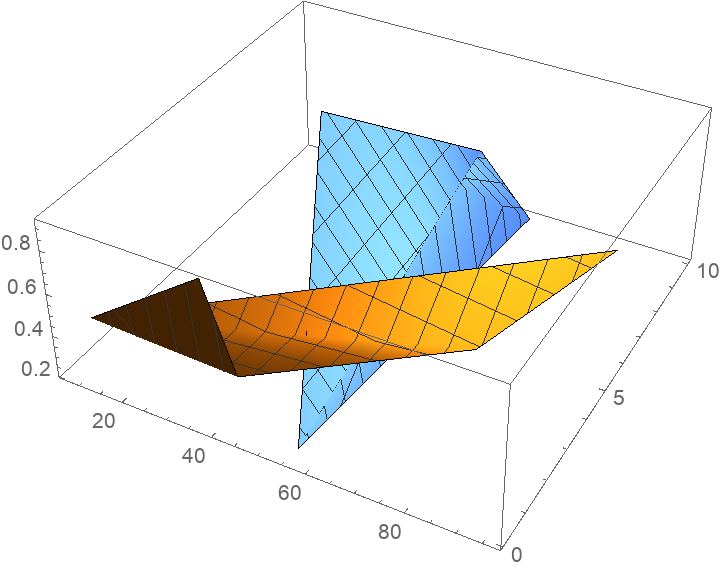## Requirements

Wolfram Language 11.3 (March 2018) or above